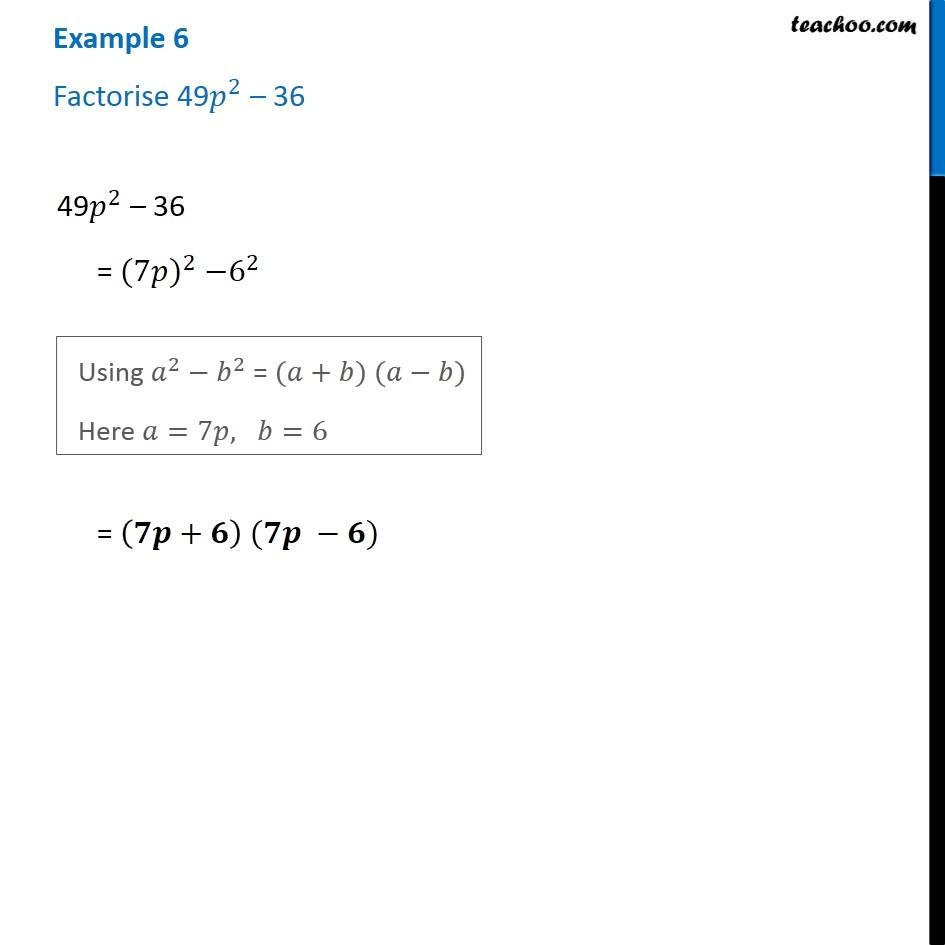1. Chapter 14 Class 8 Factorisation
2. Serial order wise
3. Examples

Transcript

Example 6 Factorise 49𝑝^2 – 36 49𝑝^2 – 36 = 〖(7𝑝)〗^2 −6^2 Using 𝑎^2−𝑏^2 = (𝑎+𝑏) (𝑎−𝑏) Here 𝑎=7𝑝, 𝑏=6 = (𝟕𝒑+𝟔) (𝟕𝒑 −𝟔)